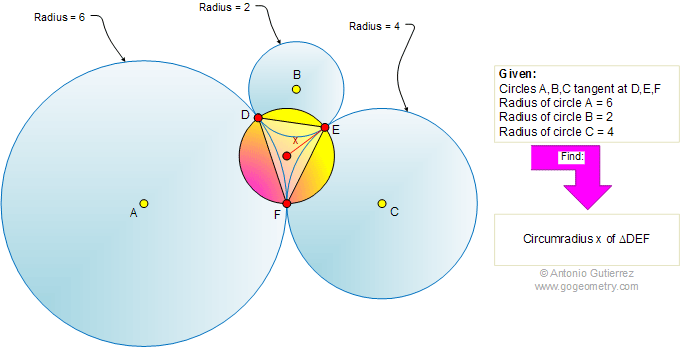# Online Geometry Problem 445: Three Tangent Circles, Tangency Points, Circumradius

 The figure shows three circles A,B, and C with radii 6, 2, and 4, respectively, each tangent to the other two at the points D, E, and F. Find the circumradius of triangle DEF. The circumradius of a triangle DEF is the radius of a circle that passes through the vertices D, E, and F.Home | Search | Geometry | Problems | All Problems | Visual Index | 441-450 | Email | Solution / comment | By Antonio Gutierrez